Latest Banking jobs   »

# Quantitative Aptitude Quiz For SBI Clerk Prelims 2022- 5th May

Directions (1-5): In each of these questions, two equations (I) and (II) are given. You have to solve both the equations and give answer
(a) if x>y
(b) if x≥y
(c) if x<y
(d) if x ≤y
(e) if x = y or no relation can be established between x and y.

Q1. I 2x² – 25x + 72 = 0
II 4y² – 12y – 27 = 0

Q2. I. x²-8x+15=0
II. y²-3y+2=0

Q3. I. 2x+3y=14
II. 4x + 2y=16

Q4. I. 2x² + 11x – 195 = 0
II. 3y² + 10y – 125 = 0

Q5. I. x² + 17x + 52 = 0
II. y² + 27y + 182 = 0

Directions (6-10): In the following questions there are two equations given. You have to solve both the equations and give answer:
(a) if x > y
(b) if x < y
(c) if x ≥ y
(d) if x ≤ y
(e) if x = y or relation between x and y cannot be established

Q6. I. x²+9x-22=0
II. 2y² – 7y + 6 = 0

Q7. I. 2y² – 13y – 34 = 0
II. 3x² – 11x – 20 = 0

Q8. I. x^4 = 256
II. y² – 16y + 64 = 0

Q9. I. x² – 46x + 528 = 0
II. y² – 48y + 572 = 0

Q10. I. 2x + 3y = 4
II. 4x + 5y = 6

Directions (11-15): In the following questions, two equations (I) and (II) are given. You have to solve both equations and mark the appropriate option.

Q11. I. x²-15x+56=0
II. y²-12y+35=0
(a) x>y
(b) x<y
(c) x≥y
(d) x≤y
(e) x=y or no relation.

Q12. I. 6x²-17x+12=0
II. 3y²-26y+48=0
(a) x>y
(b) x<y
(c) x≥y
(d) x≤y
(e) x=y or no relation.

Q13. I. (x+4)²+x+4=0
II. (y+5)²=4
(a) x>y
(b) x<y
(c) x≥y
(d) x≤y
(e) x=y or no relation.

Q14. I. (x+21)²=1681
II. (y+25)²=1849
(a) x>y
(b) x<y
(c) x≥y
(d) x≤y
(e) x=y or no relation.

Q15. I. x² – x – 132 = 0
II. y² – 22y + 112 = 0
(a) x>y
(b) x<y
(c) x≥y
(d) x≤y
(e) x=y or no relation.

Solutions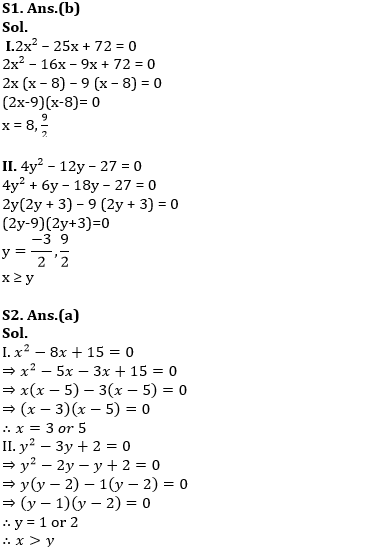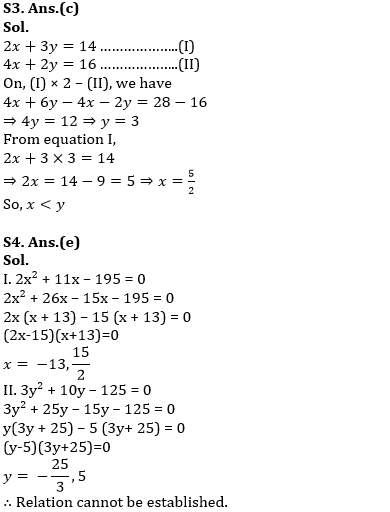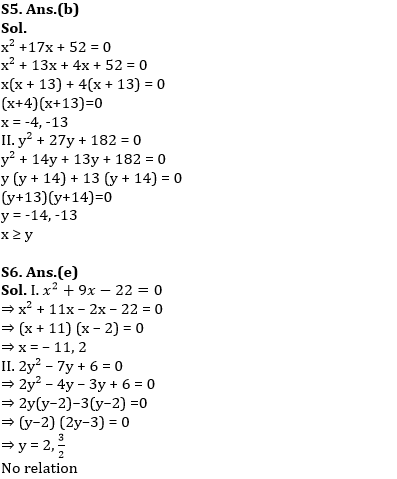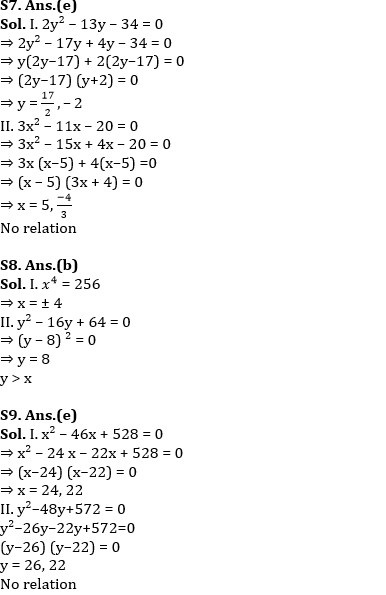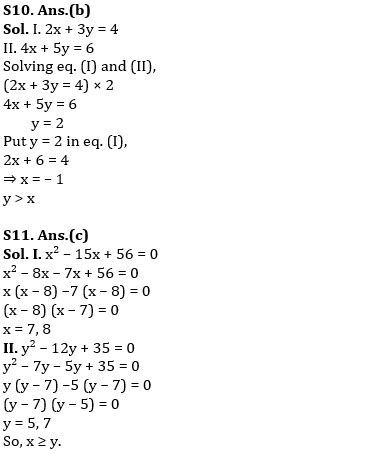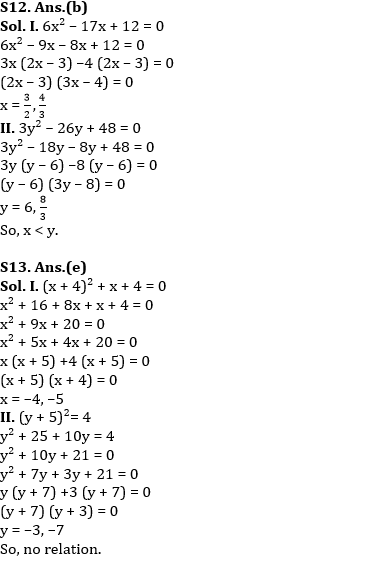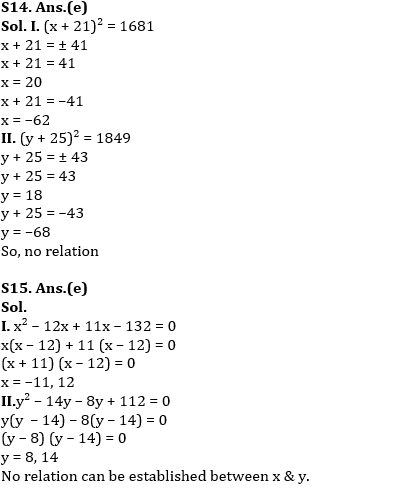#### Congratulations!

Incorrect details? Fill the form again here

•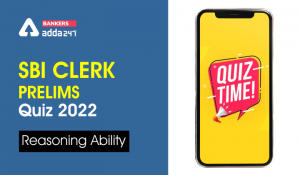Reasoning Ability Quiz For SBI Clerk Pre...
•English Quizzes For SBI Clerk Prelims 20...
•Reasoning Ability Quiz For SBI Clerk Pre...
•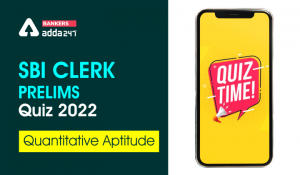Quantitative Aptitude Quiz For SBI Clerk...
•English Quizzes For SBI Clerk Prelims 20...
•Reasoning Ability Quiz For SBI Clerk Pre...